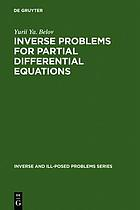Inverse problems for partial differential equations (Book, 2002) [WorldCat.org]Preview this item
Checking...

# Inverse problems for partial differential equations

Author: Ju Ja Belov Utrecht Boston Köln Tokyo VSP 2002 Inverse and ill-posed problems series.Print book : EnglishView all editions and formats This monograph is devoted to identification problems of coefficients in equations of mathematical physics. Differential properties of the solutions for the original direct problems and their behavior  Read more... (not yet rated) 0 with reviews - Be the first.

## Find a copy in the libraryFinding libraries that hold this item...

## Details

Document Type: Book Ju Ja Belov Find more information about: Ju Ja Belov 9067643580 9789067643580 248450720 Literaturverz. S. 201 - 211 VIII, 211 S. 25 cm Ch. 1. Auxiliary information from functional analysis and theory of differential equations. 1.1. The basic notions and notations. 1.2. Inequalities. 1.3. Some concepts and theorems of functional analysis. 1.4. Linear partial differential equation of the first order. 1.5. The maximum principle for parabolic equations of second order -- Ch. 2. The weak approximation method. 2.1. Examples reducing to the concept of the weak approximation method. 2.2. General formulation of the weak approximation method. 2.3. Two theorems. 2.4. An example. The linear partial differential equation -- Ch. 3. Identification problems for parabolic equations with Cauchy data. 3.1. The unknown source function. 3.2. The unknown lowest coefficient. 3.3. The unknown coefficient to the first order derivative. 3.4. An unknown coefficient to the time derivative. 3.5. Inverse problem for a semilinear parabolic equation. 3.6. Equations of Burgers type. 3.7. The splitting of one many-dimensional inverse problem into problems of lower dimension -- Ch. 4. The identification of the source function for a system of composite type and parabolic equation. The behavior of the problem's solution under t [approaches +infinity]. 4.1. The statement of the problem. 4.2. Theorems of existence and uniqueness "on the whole" 4.3. The behavior of solution by t [approaches +infinity]. 4.4. Stationary problem. 4.5. Convergence to the stationary problem solution. 4.6. Unique solvability of the problem of identifying the source function for a parabolic equation. 4.7. The stabilization of the solution to the inverse problem -- Ch. 5. The problem of determining the coefficient in a parabolic equation and some properties of its solution. 5.1. Statement of the problem. 5.2. Theorems of existence and uniqueness "on the whole" 5.3. Properties of solution under t[approaches +infinity] -- Ch. 6. Two unknown coefficients of a parabolic type equation. 6.1. Uniform boundary conditions. 6.2. Inhomogeneous conditions of over-determination. 6.3. Input data of the special form -- Ch. 7. Some inverse boundary value problems. 7.1. Unknown source function. 7.2. Nonlinear heat equation. 7.3. Hyperbolic equation with small parameter Inverse and ill-posed problems series. Yu. Ya. Belov

## Reviews

User-contributed reviewsBe the first.

## Similar Items

### Related Subjects:(6)

Confirm this request

You may have already requested this item. Please select Ok if you would like to proceed with this request anyway.